# Grouping of the cell's|Wheat stone bridge

## (6)Grouping of the cell's

• A limited ammount of current can be drawn from a single cell or battery
• There are situations where single cell fails to meet the current requirement in a circuits
• To overcome the problem cells can be grouped in series and in parallel combinations or mixed grouping of cells is done in order to obtain a large value
of electric current

(A) Series combination
• Figure below shows the two cells of emf's E1 and E2 and internal resistance r1 and r2 respectively connected in series combination through external resistance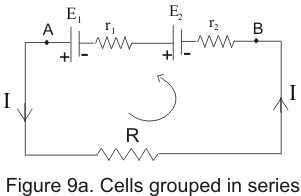• Points A and B in the circuit acts as two terminals of the combination
• Applying kirchoff's loop rule to above closed circuit
-Ir2-Ir1-IR+E1+E2=0
or
I=E1+E2/R+(r1+r2)
Where I is the current flowing through the external resistance R
• Let total internal resistance of the combination by r=r1+r2 and also let E=E1+E2 is the total EMF of the two cells
• Thus this combination of two cells acts as a cell of emf E=E1+E2 having total internal resistance r=r1+r2 as shown above in the figure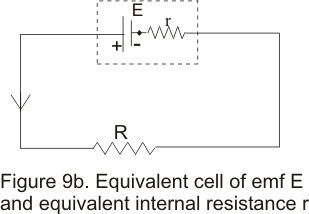(B) Parallel combinations of cells
• Figure below shows the two cells of emf E1 and E2 and internal resistance r1 and r2 respectively connected in parallel combination through external resistance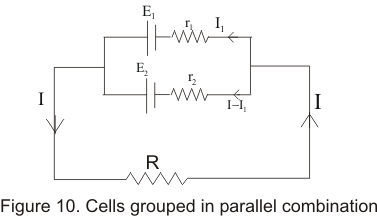• Applying kirchoff's loop rule in loop containing E1 ,r1 and R,we find
E1-IR-I1r1=0 ------------------------(1)
Similarly applying kirchoff's loop rule in loop containing E2 ,r2 and R,we find
E2-IR-(I-I1)r2=0 ------------------------(2)
• Now we have to solve equation 1 and 2 for the value of I,So multiplying 1 by r2 and 2 by r1 and then adding these equations results in following equation
IR(r1+r2)+r2r1I-E1r2-E2r1=0
which gives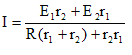We can rewrite this as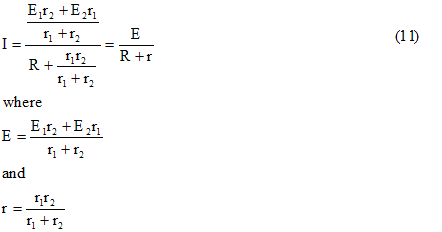E is the resulting EMF due to parallel combination of cells and r is resulting internal resistance.

(7) Wheat stone bridge
• Wheat stone bridge was designede by british physicist sir Charles F wheatstone in 1833
• It is a arrangement of four resistors used to determine resistance of one resistors in terms of other three resistors
• Consider the figure given below which is an arrangement of resistors and is knowns as wheat stone bridge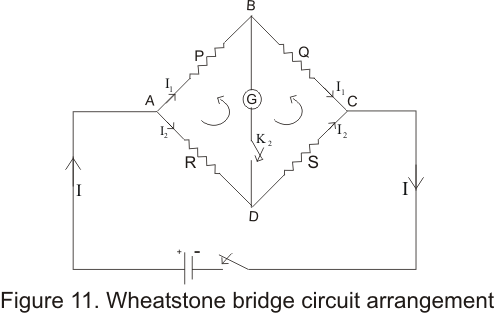• Wheatstone bridge consists of four resistance P,Q,R and S with a battery of EMF E.Two keys K1 and K2 are connected across terminals A and C and B and D respectively
• ON pressing key K1 fisrt and then pressing K2 next if galvanometer does not show any deflection then wheatstone bridge is said to be balanced
• Galavanometer is not showing any deflection this means that no current is flowing through the galvanameter and terminal B and D are at the same
potential .THus for a balanced bridge
VB=VD
• Now we have to find the condition for the balanced wheatstone bridge .For this applying kirchoff's loop rule to the loop ABDA ,we find the relation
-I2R+I1P=0
or I1P=I2R --(a)
Again applying kirchoff's rule to the loop BCDB
I1Q-I2S=0
or I1Q=I2S --(b)
From equation a and b we get
I1/I2=R/P=S/Q
or
P/Q=R/S                       (12)
• equation 12 gives the condition for the balanced wheatstone bridge
• Thus if the ratio of the resistance R is known then unknown resistance S can easily be calculated
• One important thing to note is that when bridge is balanced positions of cell and galvanometer can be exchanged without having any effect on the balance of the bridge
• Sensitivity of the bridge depends on the relative magnitudes of the resistance in the four arm of the bridge is maximum for same order of four resistance.